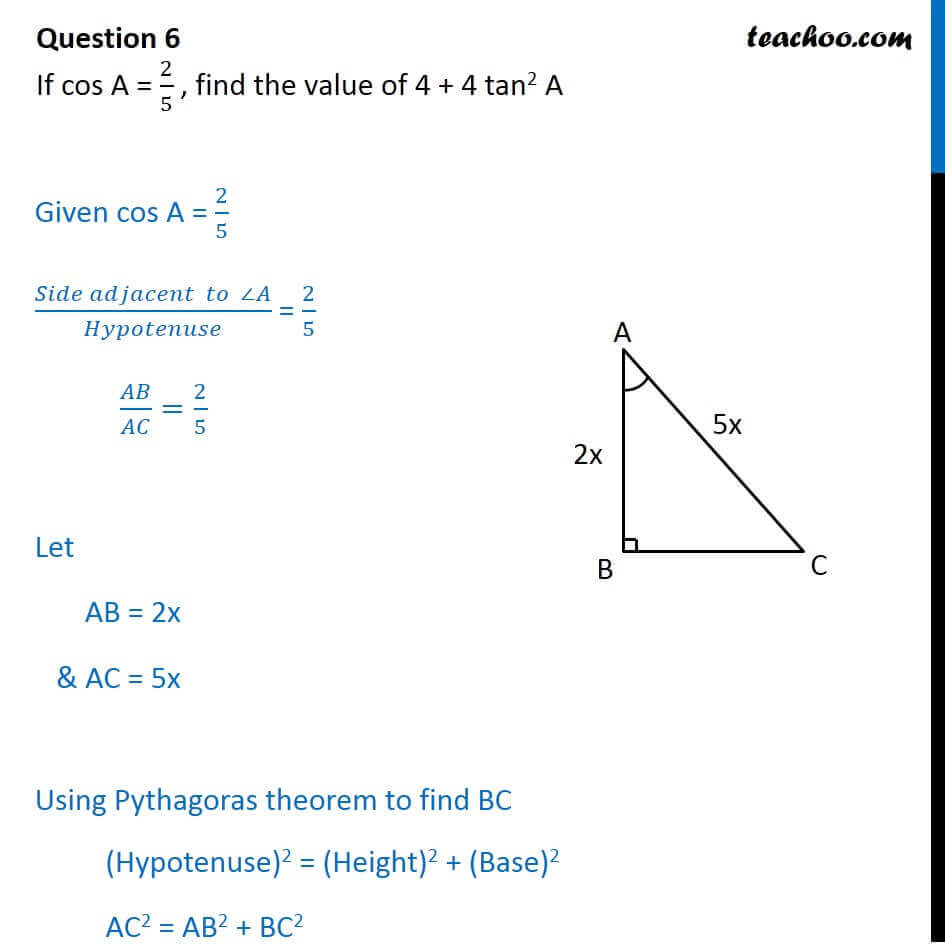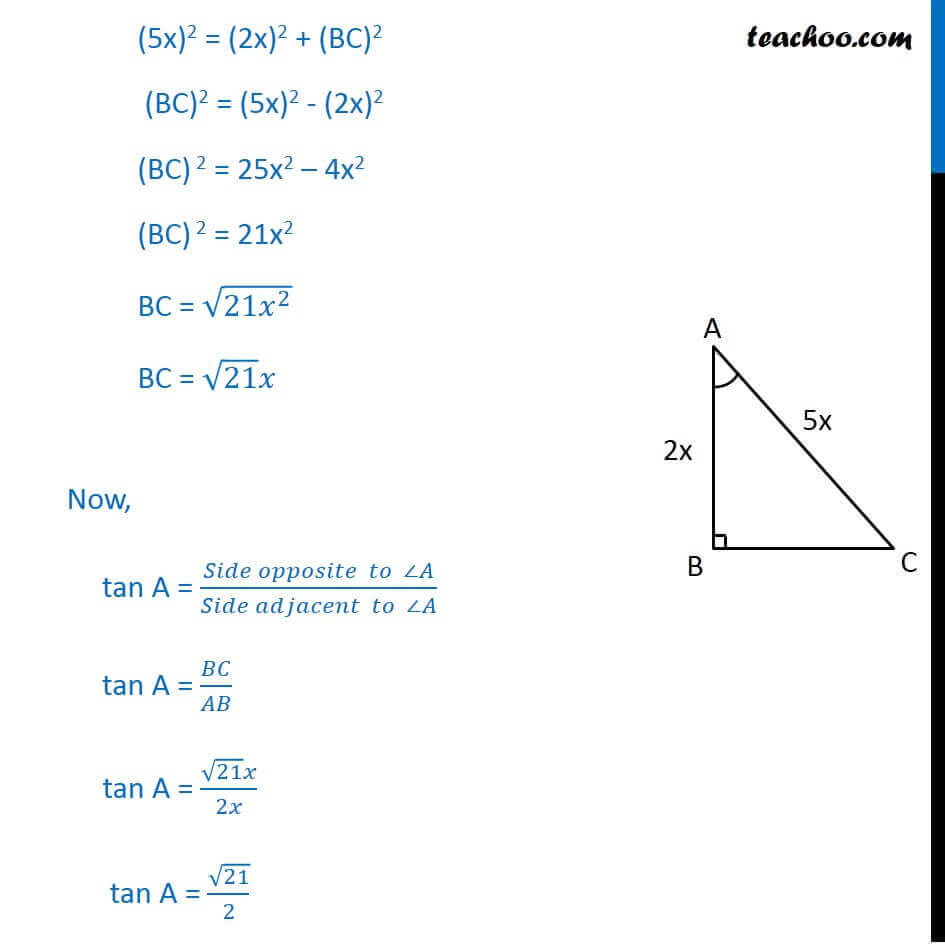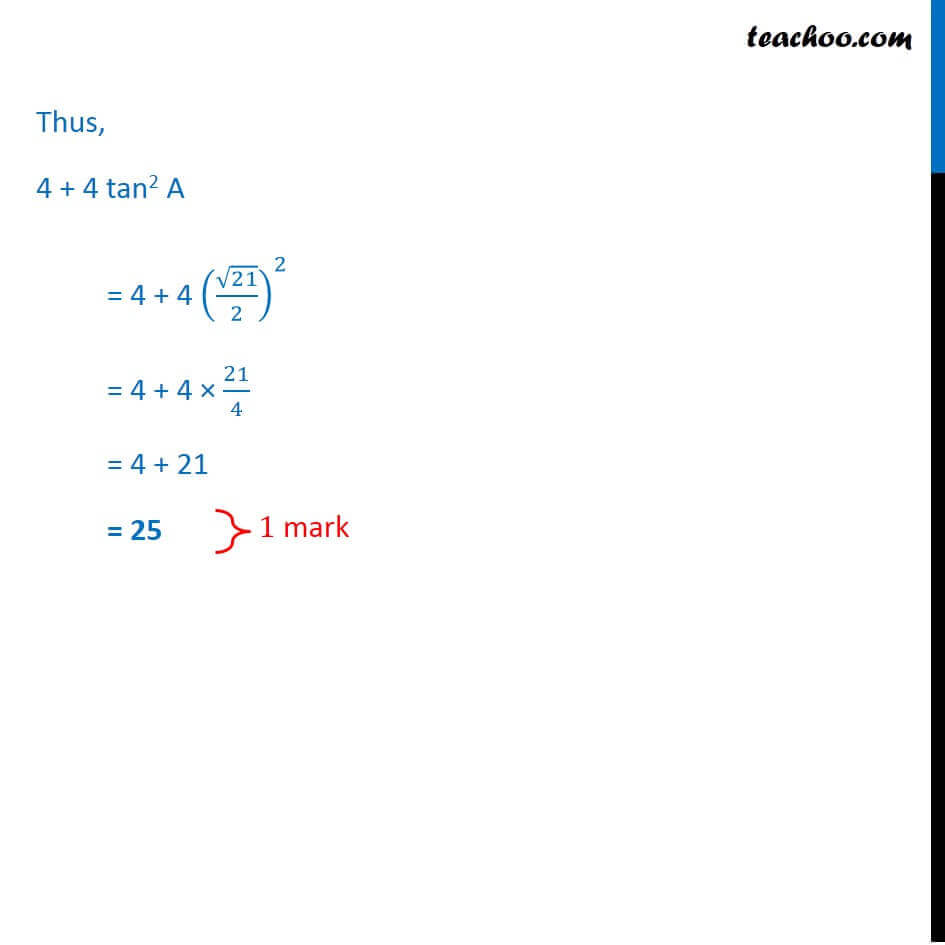CBSE Class 10 Sample Paper for 2018 Boards

Class 10
Solutions of Sample Papers for Class 10 Boards

### If cos A = 2/5 , find the value of 4 + 4 tan 2 A

This is a question of CBSE Sample Paper - Class 10 - 2017/18.Learn in your speed, with individual attention - Teachoo Maths 1-on-1 Class

### Transcript

Question 6 If cos A = 2/5 , find the value of 4 + 4 tan2 A Given cos A = 2/5 (𝑆𝑖𝑑𝑒 𝑎𝑑𝑗𝑎𝑐𝑒𝑛𝑡 𝑡𝑜 ∠𝐴)/𝐻𝑦𝑝𝑜𝑡𝑒𝑛𝑢𝑠𝑒 = 2/5 𝐴𝐵/𝐴𝐶=2/5 Let AB = 2x & AC = 5x Using Pythagoras theorem to find BC (Hypotenuse)2 = (Height)2 + (Base)2 AC2 = AB2 + BC2 (5x)2 = (2x)2 + (BC)2 (BC)2 = (5x)2 - (2x)2 (BC) 2 = 25x2 – 4x2 (BC) 2 = 21x2 BC = √(21𝑥^2 ) BC = √21 𝑥 Now, tan A = (𝑆𝑖𝑑𝑒 𝑜𝑝𝑝𝑜𝑠𝑖𝑡𝑒 𝑡𝑜 ∠𝐴)/(𝑆𝑖𝑑𝑒 𝑎𝑑𝑗𝑎𝑐𝑒𝑛𝑡 𝑡𝑜 ∠𝐴) tan A = 𝐵𝐶/𝐴𝐵 tan A = (√21 𝑥)/2𝑥 tan A = √21/2 Thus, 4 + 4 tan2 A = 4 + 4 (√21/2)^2 = 4 + 4 × 21/4 = 4 + 21 = 25